# Teaching Fractions in Middle School: Using Models

## Reviewing the Standards and Teaching Fractions with ModelsWelcome to Week 2 of the teaching fractions series. Last week, we talked about basic meanings, a little bit about fraction models, and suggested a couple ways to work fraction basics into everyday instruction.This week we’ll take a look at the progression of fraction skills through the grades before our students reach us in upper elementary and middle school math class. And then we’ll look at teaching fractions with models:-)

As upper elementary and middle school math teachers, we know our students have had fraction instruction – what fractions are, identifying fractions of shapes, finding equivalent fractions, comparing fractions, and some fraction operations; but we may not know exactly what students did in which grades.So, for a ‘quick’ overview (based on the Common Core Standards), in the earlier grades (1st – 4th ) students should be learning/mastering the following:
• students partition circles and rectangles into two and four ‘shares’ and use the terms: halves, fourths, quarters, half of, fourth of, quarter of.

• students partition rectangles into rows and columns of ‘same-size square’ and count to find the total number
• students again partition circles and rectangles into 2 and 4 parts, but add on partitioning into 3 parts. They continue to use halves, half of, etc and add on thirds and a third of.
• students should be looking at wholes and identifying those as two halves, three thirds and four fourths.

In 3rd grade math, there’s a lot more going on!:

• students partition shapes into parts with equal areas, and identify each part as a unit fraction (1/3,1/4,1/2, etc)
• students begin to develop an understanding of fractions as numbers, represent fractions on a number line., and “understand two fractions as equivalent if they’re the same size, or the same point on a number line.”
• students recognize and generate simple equivalent fractions and should also be explaining why the fractions are equivalent
• students express whole numbers as fractions, like 5/1 = 5
• students compare two fractions with the same numerator or the same denominator ‘by reasoning about their size’

By 4th grade math, students should be doing SO many of the fraction things. I’m not going to list out each skill, but the general goals of fractions in 4th grade common core are:

• extend understanding of fraction equivalence and ordering
• comparing fractions with different numerators and denominators by using common denominators or benchmarks
• build fractions from unit fractions (includes understanding addition and subtraction as joining and separating parts, as well as decomposing fractions into sums of fractions)
• add and subtract mixed #s with like denominators (replacing each mixed number with an equivalent fraction)
• solve word problems w/addition and subtraction
• multiply fraction by a whole number and understand that a/b (like 5/4) is a multiple of 1/b (¼)
• solve word problems involving multiplication of a fraction by a whole number
• understand decimal notation for fractions, and compare decimal fractions

I think it’s important to  note that ‘using visual models’ is included in numerous places in these standards.

## Fractions in 5th Grade Math (and beyond)

​By the time students get to us in 5th or 6th grade (or beyond),  students should understand all of the 1st-4th grade fraction concepts. Unfortunately, it often isn’t the case……for so many reasons.
• Some students aren’t developmentally ready for all the fraction concepts
• Some classes may not have gotten as far in the curriculum as they needed to and may never have discussed certain fraction topics
• OR they did discuss the fraction topics, but very quickly or maybe with shortcuts, because there just wasn’t enough time

So that’s all well and good….students are supposed to have done all of this, should be proficient at these fraction skills, and should be ready to apply them and go deeper at the next grade level.

But what are math teachers at 5th, 6th, or higher grade levels supposed to do when this isn’t the case, and their math curriculum builds on that prior fraction knowledge? The ‘time’ issue continues to be an issue. Where does reteaching fit into the curriculum? (A question for many math concepts, but we’ll stick to fractions here!)

## Teaching Fractions with Models in Middle School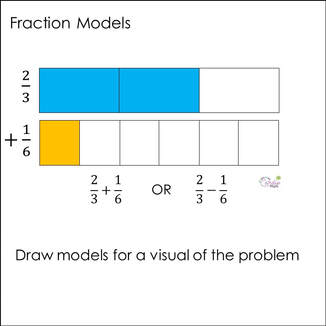I don’t have all the answers, but I have a few ideas.
Let’s look at how using models might be able to help. Based on the standards, student should have been using various models before hitting 5th grade – area models, length models, set models, and number lines. Continuing to use these fraction models will only help students connect the concepts with the processes.

One way to help students bridge some of the fraction gaps (or just refresh their memories) is to incorporate these visual models as much as possible.
When teaching a new fraction concept (or briefly reviewing concepts from previous years), bring in the models.

For example, when teaching adding or subtracting fractions with unlike denominators (2/3 + 1/6 or 2/3 – 1/6), include a model next to the problem or have students draw models.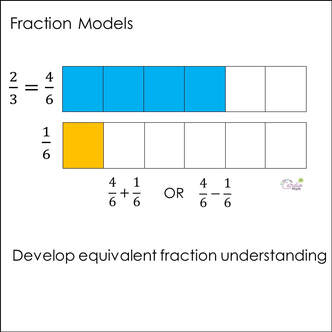• On their models, students can add partitions to show both fractions as sixths, encouraging the continued development of equivalent fractions.

• The addition and subtraction of fractional parts is visual for middle school students when they use models.

This combination of process and modeling helps students connect the process with the meaning of the operations, or of simply finding equivalent fractions.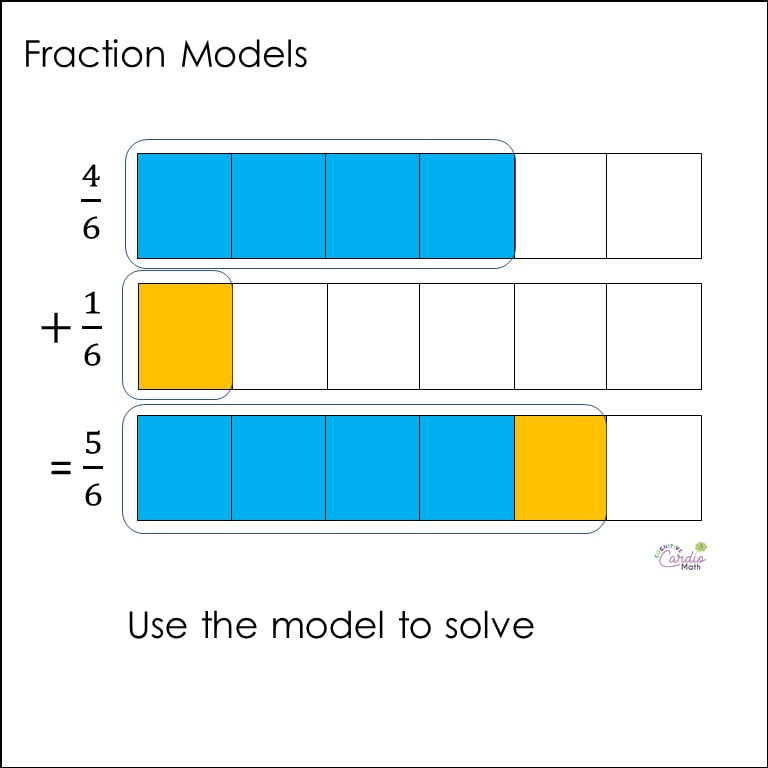## Other Fraction Models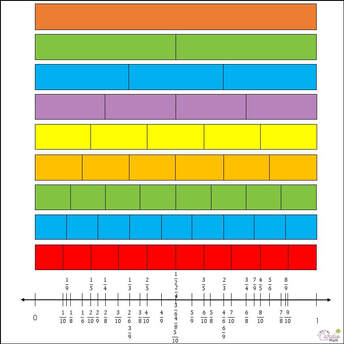As students get into higher grade levels, the length model is extremely helpful, since it’s easy to transfer to a number line.

While you probably don’t want to redraw the lengths and the number line for every new problem, you can create and post some length/number line models around the classroom and provide models students can refer to on a regular basis.This can again reinforce the concept of equivalent fractions, help students with comparing fractions, and transfer to fraction, decimal equivalences.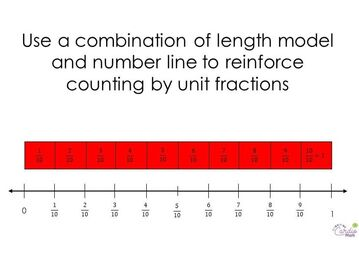Length models, aligned with a number line, can also be helpful to reinforce the idea that students can count by unit fractions the same way they can count  by whole numbers.

## Fraction Toolkit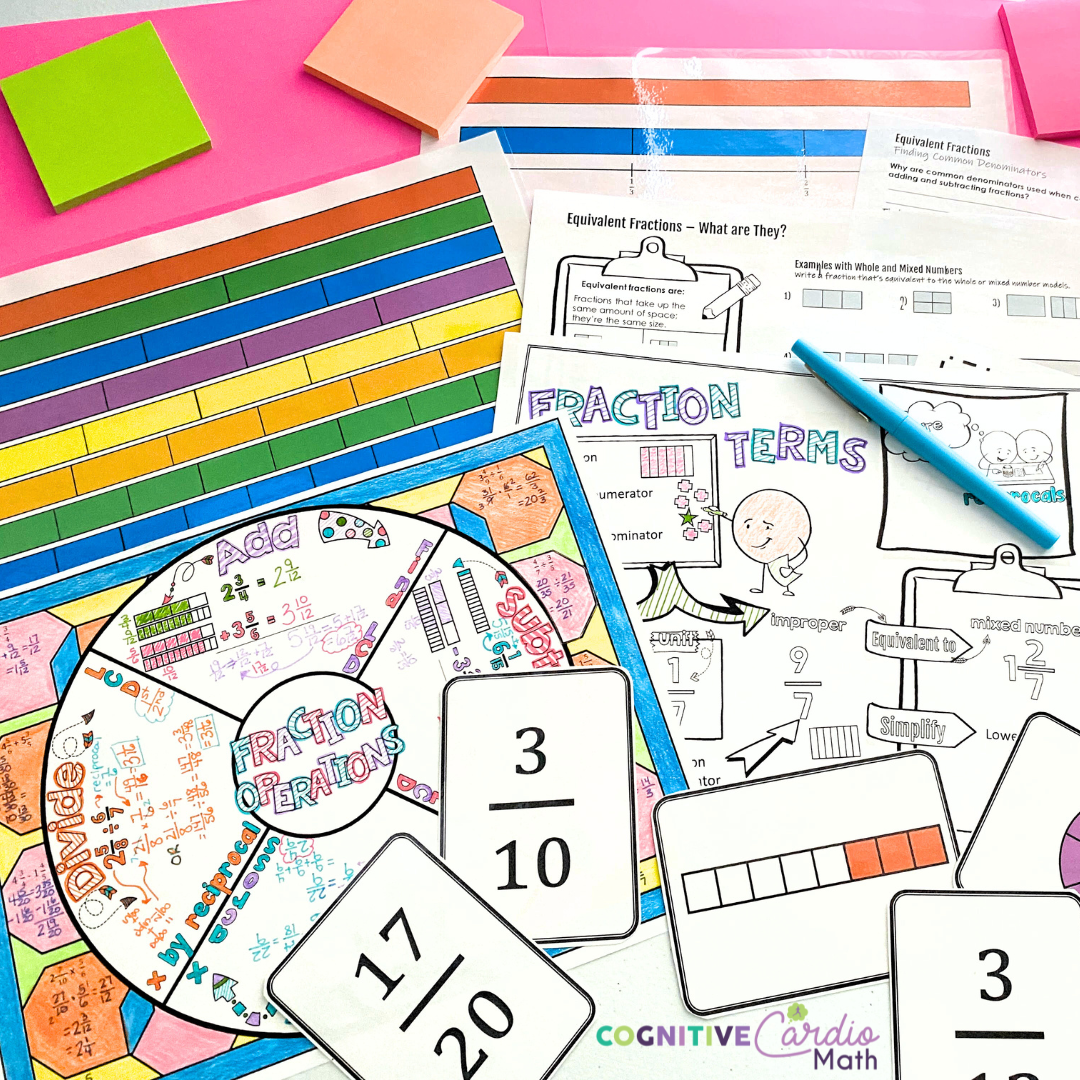Do you need a few tools to help your students master the fraction basics? I’ve got a ‘Fraction Toolkit’ for you!

You can grab the Fraction Toolkit and learn how to use it in this post

## EllieWelcome to Cognitive Cardio Math! I’m Ellie, a wife, mom, grandma, and dog ‘mom,’ and I’ve spent just about my whole life in school! With nearly 30 years in education, I’ve taught:

• All subject areas in 4th and 5th grades
• Math, ELA, and science in 6th grade (middle school)

I’ve been creating resources for teachers since 2012 and have worked in the elearning industry for about five years as well!